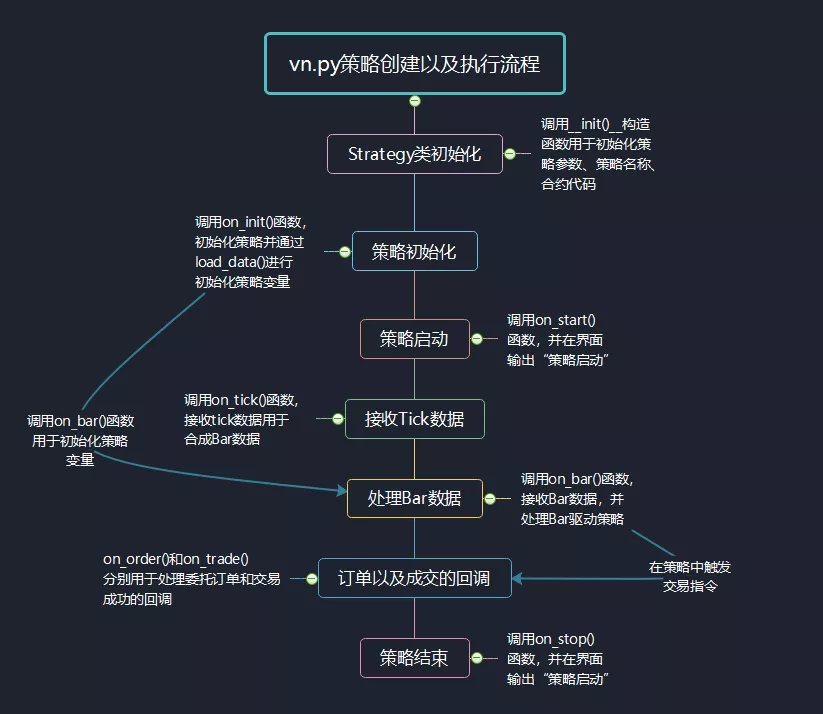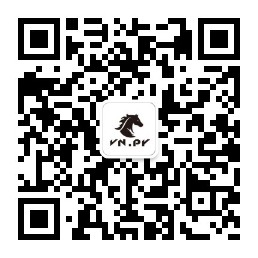### 策略原理

• 当短周期均线从下往上突破长周期均线，也就意味着当前时间段具有上涨趋势，突破点也就是常说的金叉，意味着多头信号；
• 当短周期均线从上向下突破短周期信号，则意味着当前时间段具有下降趋势，突破点也就是常说的死叉，意味着空头信号。

### 源码分析

1、创建策略实例

``````class DoubleMaStrategy(CtaTemplate):
author = "用Python的交易员"

fast_window = 10
slow_window = 20

fast_ma0 = 0.0
fast_ma1 = 0.0

slow_ma0 = 0.0
slow_ma1 = 0.0

parameters = ["fast_window", "slow_window"]
variables = ["fast_ma0", "fast_ma1", "slow_ma0", "slow_ma1"]

def __init__(self, cta_engine, strategy_name, vt_symbol, setting):
""""""
super(DoubleMaStrategy, self).__init__(
cta_engine, strategy_name, vt_symbol, setting
)

self.bg = BarGenerator(self.on_bar)
self.am = ArrayManager()``````

2、状态变量初始化

``````def on_init(self):
"""
Callback when strategy is inited.
"""
self.write_log("策略初始化")

``````def load_bar(
self,
days: int,
interval: Interval = Interval.MINUTE,
callback: Callable = None,
):
"""
Load historical bar data for initializing strategy.
"""
if not callback:
callback = self.on_bar       #设置回调函数

``````def load_bar(
self,
vt_symbol: str,
days: int,
interval: Interval,
callback: Callable[[BarData], None]
):
""""""
symbol, exchange = extract_vt_symbol(vt_symbol)
end = datetime.now()
start = end - timedelta(days)

bars = self.query_bar_from_rq(symbol, exchange, interval, start, end)
if not bars:
symbol=symbol,
exchange=exchange,
interval=interval,
start=start,
end=end,
)

for bar in bars:
callback(bar)``````

3、启动自动交易

def on_start(self):

``````    """
Callback when strategy is started.
"""
self.write_log("策略启动")
self.put_event()``````

4、接收Tick推送

``````def on_tick(self, tick: TickData):
"""
Callback of new tick data update.
"""
self.bg.update_tick(tick)``````

``````def update_tick(self, tick: TickData):
"""
Update new tick data into generator.
"""
new_minute = False

# Filter tick data with 0 last price
if not tick.last_price:
return

if not self.bar:
new_minute = True
elif self.bar.datetime.minute != tick.datetime.minute:
self.bar.datetime = self.bar.datetime.replace(
second=0, microsecond=0
)
self.on_bar(self.bar)
new_minute = True

if new_minute:
self.bar = BarData(
symbol=tick.symbol,
exchange=tick.exchange,
interval=Interval.MINUTE,
datetime=tick.datetime,
gateway_name=tick.gateway_name,
open_price=tick.last_price,
high_price=tick.last_price,
low_price=tick.last_price,
close_price=tick.last_price,
open_interest=tick.open_interest
)
else:
self.bar.high_price = max(self.bar.high_price, tick.last_price)
self.bar.low_price = min(self.bar.low_price, tick.last_price)
self.bar.close_price = tick.last_price
self.bar.open_interest = tick.open_interest
self.bar.datetime = tick.datetime

if self.last_tick:
volume_change = tick.volume - self.last_tick.volume
self.bar.volume += max(volume_change, 0)

self.last_tick = tick``````

update_tick函数内部主要是通过检查当前的Tick数据与上一笔Tick数据是否是属于同一分钟，来判断是否有新的1分钟K线生成，如果没有就会继续进行累加更新当前K线的信息。

5、核心交易逻辑

• 如果策略逻辑是基于Tick数据的，则在on_tick函数中实现相关的交易逻辑；
• 如果策略逻辑是基于K线的，如这里我们的双均线策略，则在on_bar函数中实现相关的交易逻辑。

``````def on_bar(self, bar: BarData):
"""Callback of new bar data update."""
am = self.am
am.update_bar(bar)
if not am.inited:
return

fast_ma = am.sma(self.fast_window, array=True)
self.fast_ma0 = fast_ma[-1]
self.fast_ma1 = fast_ma[-2]

slow_ma = am.sma(self.slow_window, array=True)
self.slow_ma0 = slow_ma[-1]
self.slow_ma1 = slow_ma[-2]

cross_over = self.fast_ma0 > self.slow_ma0 and self.fast_ma1 < self.slow_ma1
cross_below = self.fast_ma0 < self.slow_ma0 and self.fast_ma1 > self.slow_ma1

if cross_over:
if self.pos == 0:
elif self.pos < 0:
self.cover(bar.close_price, 1)

elif cross_below:
if self.pos == 0:
self.short(bar.close_price, 1)
elif self.pos > 0:
self.sell(bar.close_price, 1)
self.short(bar.close_price, 1)

self.put_event()``````

``````    am = self.am
am.update_bar(bar)
if not am.inited:
return

fast_ma = am.sma(self.fast_window, array=True)
self.fast_ma0 = fast_ma[-1]
self.fast_ma1 = fast_ma[-2]

slow_ma = am.sma(self.slow_window, array=True)
self.slow_ma0 = slow_ma[-1]
self.slow_ma1 = slow_ma[-2]``````

``````    cross_over = self.fast_ma0 > self.slow_ma0 and self.fast_ma1 < self.slow_ma1
cross_below = self.fast_ma0 < self.slow_ma0 and self.fast_ma1 > self.slow_ma1``````

• 当出现了金叉：如果没有持仓时，则直接买入开仓；或者持有空头，则先平空再买入开仓。
• 当出现了死叉：如果没有持仓时，则直接卖出开仓；或者是持有多头，则先平多再卖出开仓。

• sell：卖出平仓（ Direction：空，Offset：平）
• short：卖出开仓（ Direction：空，Offset：开）
• cover：买入平仓（ Direction：多，Offset：平）

``````if cross_over:
if self.pos == 0:
elif self.pos < 0:
self.cover(bar.close_price, 1)

elif cross_below:
if self.pos == 0:
self.short(bar.close_price, 1)
elif self.pos > 0:
self.sell(bar.close_price, 1)
self.short(bar.close_price, 1)

self.put_event()``````

6、委托回报

on_order是委托回调函数，当我们发出一个交易委托后，这个委托每当有状态变化时，我们都会收到该委托最新的数据推送，这条数据就是委托回报。

``````def on_order(self, order: OrderData):
"""
Callback of new order data update.
"""
pass``````

7、停止自动交易

``````def on_stop(self):
"""
Callback when strategy is stopped.
"""
self.write_log("策略停止")
self.put_event()``````

### CTA交易流程梳理《vn.py全实战进阶》课程全新上线，一共50节内容覆盖从策略设计开发、参数回测优化，到最终实盘自动交易的完整CTA量化业务流程，目前已经更新到第八集，详细内容请戳课程上线：《vn.py全实战进阶》！